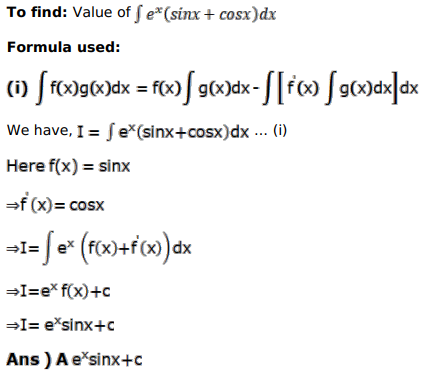# Mark against the correct answer in each of the following:

Question:

Mark $(\sqrt{)}$ against the correct answer in each of the following:

$\int e^{x}(\sin x+\cos x) d x=?$

A. $e^{x} \sin x+C$

B. $e^{x} \cos x+C$

C. $e^{x} \tan x+C$

D. None of these

Solution: